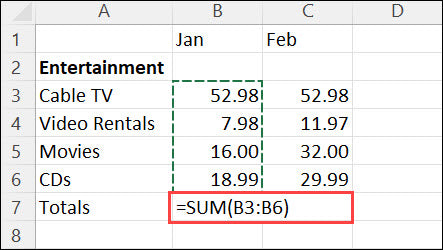Blog

# How to Make a Formula in Excel?

If you’re looking for a way to save time and automate tedious tasks in Excel, then learning how to create a formula is the perfect solution. Excel formulas are powerful tools that allow you to quickly calculate results and make decisions based on data. In this guide, we’ll provide you with an easy step-by-step tutorial on how to make a formula in Excel. You’ll learn how to identify and use the right formula, as well as how to troubleshoot and debug any errors that might occur. So, let’s get started!## Creating a Formula in Excel

Creating a formula in Excel is a great way to quickly calculate data in a spreadsheet. Excel formulas can be used to perform a variety of calculations, from simple arithmetic to complex financial analysis. With a few simple steps, you can easily create a formula in Excel.

### Step 1: Open the Excel Worksheet

The first step to creating a formula in Excel is to open the Excel worksheet. This can be done by double-clicking the Excel file on your computer or by selecting the “File” menu and then selecting “Open”. Once the Excel file is open, you will be able to see the spreadsheet with the data that you want to use in the formula.

### Step 2: Select the Cell for the Formula

The next step is to select the cell where the formula will be entered. This can be done by clicking on the cell or by using the arrow keys to move the cursor to the cell. Once the cell is selected, the cell will be highlighted in blue.

### Step 3: Enter the Formula

After selecting the cell, you can begin to enter the formula. To do this, type an equal sign (=) followed by the formula. The formula should include the cell references for the data that you want to use in the calculation. For example, if you want to calculate the sum of two cells, you would enter “=A1+B1”.

### Step 4: Check the Formula

Once the formula is entered, it is important to check it to make sure that it is correct. To do this, you can use the “Check Formula” option in the “Formula” menu. This will check the formula for any errors and will alert you if any errors are found.

### Step 5: Enter the Formula

Once the formula has been checked and is correct, you can enter the formula by pressing the “Enter” key. This will calculate the formula and display the result in the cell. If the formula is correct, the result will be displayed in the cell.

### Step 6: Modify the Formula

If the formula needs to be modified, you can do so by selecting the cell and then editing the formula. After making the changes, you should check the formula again to make sure that it is correct. Once the formula is correct, you can enter the formula again by pressing the “Enter” key.

### Step 7: Save the Worksheet

Finally, after entering the formula, it is important to save the worksheet. This can be done by selecting the “File” menu and then selecting “Save”. This will save the worksheet with the formula that you have entered.

## Related Faq

### What is a Formula in Excel?

A formula in Excel is a function or expression that is used to perform a calculation or generate a result. It can be used to add, subtract, multiply, or divide data from cells, perform logical tests, and other complex calculations. Formulas are written using the equals sign (=) followed by the formulas components, such as cell references, mathematical operators, and functions.

### What are the Different Types of Formulas?

The two main types of formulas in Excel are mathematical formulas and logical formulas. Mathematical formulas are used to perform mathematical calculations such as adding, subtracting, multiplying, and dividing. Logical formulas are used to perform logical tests such as comparing two values, determining whether a value is greater than or less than another value, or determining if a value is equal to a certain value.

### How Do You Create a Formula in Excel?

Creating a formula in Excel is simple. First, enter the equals sign (=) followed by the cells, operators, and functions you want to use in the formula. Then, press enter to execute the formula. The result of the formula will be displayed in the cell.

### What Are Some Examples of Formulas in Excel?

A few examples of formulas in Excel are:
• Subtracting two cells: =A1-B1
• Multiplying two cells: =A1*B1
• Dividing two cells: =A1/B1
• Finding the maximum of two cells: =MAX(A1,B1)
• Finding the minimum of two cells: =MIN(A1,B1)

### What Are the Different Operators Used in Formulas?

There are several operators used in formulas, such as addition (+), subtraction (-), multiplication (*), division (/), and exponentiation (^). Additionally, there are comparison operators such as greater than (>), less than (). There are also logical operators such as AND, OR, and NOT.

### What is the Difference Between Formulas and Functions?

Formulas and functions are both types of expressions used in Excel. The difference is that formulas are used to perform calculations, while functions are pre-defined formulas that perform specific calculations. Functions can be used to calculate the sum, average, minimum, maximum, and other complex calculations.

### Excel Formulas and Functions Tutorial

If you want to become an Excel pro and make your life easier, learning how to make a formula in Excel is essential. With the right knowledge and practice, you can easily apply formulas with confidence and accuracy. Through understanding the basics of Excel formulas, you can save time and increase your efficiency. With the right skillset, you can create powerful spreadsheets and simplify complex calculations. Excel formulas can turn tedious and time-consuming tasks into a breeze.